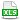HomeTechniques and TipsCorrelation in @RISKHow @RISK Correlates Inputs

# 5.1. How @RISK Correlates Inputs

Applies to: @RISK, all releases

How do I specify correlations?

When two or more input variables should be correlated, you can click the Define Correlations icon in the ribbon, specify correlations in the Model Definition Window, or add RiskCorrmat( ) functions directly to the distribution formulas for those variables in your Excel sheet.

The correlation coefficients you specify are Spearman rank-order correlations, not Pearson linear correlations. The rank-order correlation coefficient was developed by C. Spearman in the early 1900's, and this article explains how @RISK computes the rank-order correlation coefficient.

Pearson correlations assume linear distributions, but the great majority of distributions are non-linear, and Spearman is usually more appropriate for non-linear distributions. A Web search for choose Spearman or Pearson correlation will show lots of articles about the different uses of these two forms of correlation.

During a simulation, how does @RISK draw random numbers to achieve my specified correlations?

@RISK draws all samples for correlated variables before the first iteration of the simulation. (Non-correlated variables are sampled within each iteration.) Knowing the number of iterations to be performed, @RISK adjusts the ranking and associating of samples within each iteration to yield the defined correlation values.  Again, this correlation is based on rankings of values, not actual values themselves as with the linear correlation coefficient. A value's "rank" is determined by its position within the min-max range of possible values for the variable.

@RISK generates rank-correlated pairs of sampled values in a two-step process:

1. A set of randomly distributed "rank scores" is generated for each variable. If 100 iterations are to be run, for example, 100 scores are generated for each variable. (Rank scores are simply values of varying magnitude between a minimum and maximum. @RISK uses van der Waerden scores based on the inverse function of the normal distribution.) These rank scores are then rearranged to give pairs of scores which generate the desired rank-order correlation coefficient. For each iteration there is a pair of scores, with one score for each variable.

2. A set of random numbers (between 0 and 1) to be used in sampling is generated for each variable. Again, if 100 iterations are to be run, 100 random numbers are generated for each variable. These random numbers are then ranked smallest to largest. For each variable, the smallest random number is then used in the iteration with the smallest rank score, the second smallest random number is used in the iteration with the second smallest rank score, and so on. This ordering based on ranking continues for all random numbers, up to the point where the largest random number is used in the iteration with the largest rank score

This process results in a set of paired random numbers that can be used in sampling values from the correlated distributions during an iteration of the simulation.

This method of correlation is known as a "distribution-free" approach because any distribution types may be correlated. Although the samples drawn for the two distributions are correlated, the integrity of the original distributions is maintained. The resulting samples for each distribution reflect the distribution function from which they were drawn.

Does @RISK use Cholesky decomposition?

Yes. If Cholesky fails, the matrix is not self-consistent, and @RISK proceeds as in How @RISK Adjusts an Invalid Correlation Matrix.

If Cholesky succeeds, @RISK proceeds as in Iman, R. L., and W. J. Conover. 1982. "A Distribution-Free Approach to Inducing Rank Correlation Among Input Variables." Commun. Statist.-Simula. Computa. 11: 311-334. Retrieved 2018-08-23 from https://www.uio.no/studier/emner/matnat/math/STK4400/v05/undervisningsmateriale/A distribution-free approach to rank correlation.pdf.

Why does Excel report a different correlation from the one I specified?

This correlation method yields a rank order correlation (Spearman coefficient) that is usually quite close (within normal statistical variability) to your specified value. However, Excel's =CORREL( ) function reports the Pearson coefficient.  The Pearson value may vary somewhat from the Spearman value, depending on the exact nature of the correlated distributions. This difference is illustrated in the attached workbook. (Beginning with @RISK 5.5, you can use the =RiskCorrel( ) worksheet function to display the Spearman or Pearson correlation for simulated data.)

Correlation of discrete distributions can be a particular problem.  For more on that, please see Correlation of Discrete Distributions.

See also: Correlation in @RISK collects over a dozen articles explaining various aspects.

•RankOrderComparison.xls